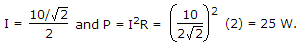# Electronics and Communication Engineering - Networks Analysis and Synthesis

1.

The number of electrons in motion at the instant of positive peak of a symmetrical ac wave is the same as that at the instant of negative peak.

 A. True B. False

Explanation:

Only directions are opposite.

2.

In figure, the current through R, after the steady state condition is reached, is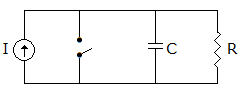A. I B. 0 C.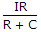D. infinite

Explanation:

At t = ∞ capacitor behaves as O.C.

3.

A two branch parallel tuned circuit has 100 Ω resistance and 0.1 H inductance in one branch and 10-6 F capacitor in the other. At resonance frequency, the dynamic resistance is

 A. 10 Ω B. 100 Ω C. 1000 Ω D. 10000 Ω

Explanation:

Dynamic resistance =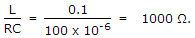4.

Special circuits using neon tubes, contact relays and capacitors are used in many domestic appliances. The role of capacitor in figure is to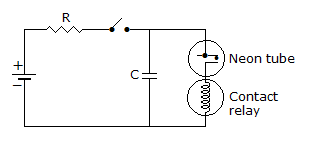A. reduce power loss B. function as resistor when relay is on C. delay the operation of the contact relay after switching on D. open circuit the relay terminals

Explanation:

No answer description available for this question. Let us discuss.

5.

A series R, L, C circuit has R = 2Ω, L = 1H, C = 1 μF. It is connected to an ac variable frequency source of peak voltage 10 V. At resonance the power supplied by the source will be

 A. 50 Ω B. 25 Ω C. 12.5 Ω D. 6.25 Ω